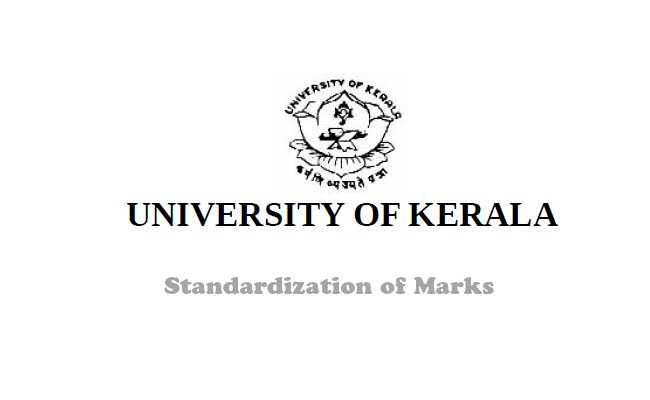Breaking News# Kerala University Mark Standardization Guidelines

Kerala University Mark Standardization Guidelines: Kerala university issued guidelines for How to standardize the Plus Two or VHSE marks for admission to First Degree Programme under CBCS in the university.

In the case of Higher Secondary Examination from 2010 onwards, the index marks are to be calculated as follows.

Total marks secured for Higher Secondary + Marks secured in the concerned subject.

For e.g. If the student has secured 800 marks out of the total 1200 marks and if he has secured 150 out of 200 in mathematics, his index marks will be 800 + 150 = 950.
In the case of admission to B.A English, double the percentage of marks obtained for English are added to the total mark. If the student in the above example has secured 120 marks out of 200 in English, his index mark will be 800 +120 = 920.

In the case of Higher Secondary Examination prior to 2010, normalize the total marks obtained out of 600 to 1200 and add twice the marks obtained in the concerned subject for calculation of index mark.

In the case of admission to B.A English, double the percentage of marks obtained for English are added the same to the total marks, normalized to 1200.

In the case of Vocational Higher Secondary examination, exclude the marks for vocational subjects and foundation course and normalize the marks as follows:

* In the case of VHSE (prior to 2010) The marks for English (out of 100) to be normalized to 200. Marks for Optional (3 subjects) (Total 3 x 100=300) to be normalized to 400.

For e.g…, if a VHSE student received the following marks,
English = 41, Optional 1 =47, Optional 2 = 58, Optional 3 = 65 Total = 211.
i. 41/100×200 for English = 82
ii. For Optionals, 170/300 x 400 = 226.66
Total out of 600 = 308.66

Total out of 1200 = 308.66 x 2 = 617.32

In the case of admission to B.A English, double the
percentage of marks in English and add to total ie.,
617.32 + 82.00 = 699.32. Index Marks is 699.32.

* In the case of VHSE students from 2010 onwards English Total = 200, Optional 1 =200, Optional 2 = 200, Optional 3 = 200. Therefore, Grand total = 800.

Normalize as follows:
i. Normalize English marks to 400
Eg: If a student received 150 marks out of 200 then, 150/200 x 400 = 300
ii.Normalize optional to 800

Eg: If a student received a total of 500 marks out of 600 in the 3 optionals, then, 500/600 x
800 = 666.66 Therefore, total out of 1200 = 966.66

In the case of admission to First Degree Programme in English, since the
marks are awarded out of 200, add these marks to the normalized optional
marks. Index marks = 966.66 +150 = 1116.66

In the case of other Boards such as CBSE and ISC, normalize the total marks to 1200 and then add TWICE THE PERCENTAGE of marks obtained in the concerned subject For Example: If a student has secured 400 out of the total of 500 marks in the CBSE stream and if he has say, secured 80 marks out of 100 in mathematics, his index marks will be 400/500 x 1200 plus 160 (i, e, 80 X 2) marks of mathematics. That is 960 + 160 =1120 In the case of admission to First Degree Programme in English, double the percentage of marks obtained for English and add to the normalized total marks.
For eg: If the student in example above secured 70 out of 100 marks in English, then his index mark will be 960 + 140 = 1100.
Weightage and Grace marks shall be given in applicable cases as laid down in the Hand Book for Admissions.

Weightage of marks for N.C.C. /N.S.S. Certificate holders/Ex-servicemen/ Dependents of Ex-service Men and Jawans
Bonus marks in respect of N.C.C. and N.S.S. candidates will be given for the purpose of ranking (Academic Council dated 25-10-1980, Syndicate dated 04-08-1980) in the manner given below:

First Degree Programme 15 marks

Resolving of tie:
In the case of tie of index marks, the following will be considered
* Marks of the subject opted
* Marks of English
* Date of Birth (older be placed higher in ranking)
* Name of the Candidate (Alphabetical order)

Loosing of marks for subsequent appearances
Candidates will lose 10 marks for ranking purpose for each additional appearance he/she has taken for completing the qualifying examination.